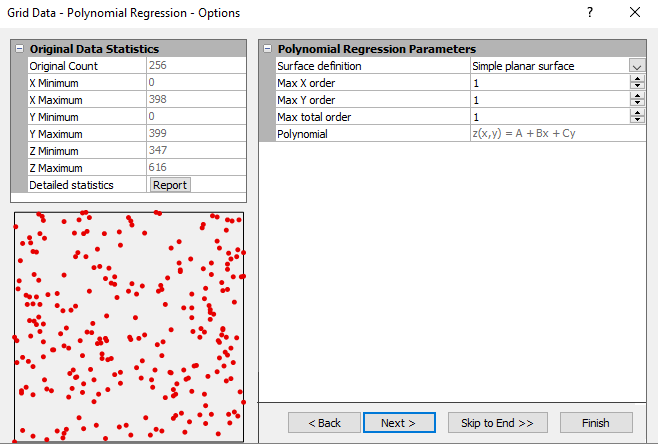# Polynomial Regression

Polynomial Regression is used to define large-scale trends and patterns in your data. Polynomial Regression is not really an interpolator because it does not attempt to predict unknown Z values. There are several options you can use to define the type of trend surface.

If you would like a report of the coefficients used in the calculation of the surface, make sure to select the Grid Report check box in the Grid Data dialog.

## Polynomial Regression Advanced Options Dialog

In the Grid Data dialog, specify Polynomial Regression as the Gridding Method and click the Next button to open the Grid Data Polynomial Regression Options dialog.Specify the Surface Definition and Parameters in the Grid Data Polynomial Regression Options dialog.

### Surface Definition

You can select the type of polynomial regression to apply to your data from the Surface Definition group. As you select the different types of polynomials, a generic polynomial form of the equation is presented in the dialog, and the values in the Parameters group change to reflect the selection. The available choices are:

• Simple planar surface
• Cubic surface
• User defined polynomial

### Parameters

The Parameters group allows you to specify the maximum powers for the X and Y component in the polynomial equation. As you change the Parameters values, the options are changed in the Surface Definition group to reflect the defined parameters.

• The Max X Order specifies the maximum power for the X component in the polynomial equation.
• The Max Y Order specifies the maximum power for the Y component in the polynomial equation.
• The Max Total Order specifies the maximum sum of the Max X Order and Max Y Order powers. All of the combinations of the X and Y components are included in the polynomial equation as long as the sum of the two powers does not exceed the Max Total Order value.

## Reference

Draper, N., and Smith, H. (1981), Applied Regression Analysis, second edition, Wiley-Interscience, 709 pp.